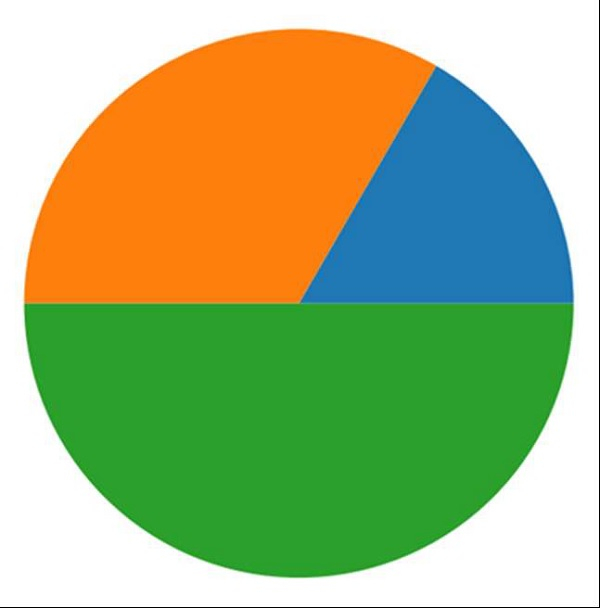# How to maximize a plt.show() window using Python?

MatplotlibServer Side ProgrammingProgrammingPython

#### Python Data Science basics with Numpy, Pandas and Matplotlib

Most Popular

63 Lectures 6 hours

#### Data Visualization using MatPlotLib & Seaborn

11 Lectures 4 hours

#### MatPlotLib with Python

9 Lectures 2.5 hours

Using plt.get_current_fig_manager() and mng.full_screen_toggle() methods, we can maximise a plot.

## Steps

• Add a subplot to the current figure, where nrow = 1, ncols = 1 and index = 1.

• Create a pie chart using list [1, 2, 3] and pie() method.

• Return the figure manager of the current figure, using get_current_fig_manager() method. The figure manager is a container for the actual backend-depended window that displays the figure on the screen.

• Create an abstract base class to handle drawing/rendering operations using the full_screen_toggle() method.

• Use plt.show() to show the figure.

## Example

import matplotlib.pyplot as plt

plt.subplot(1, 1, 1)
plt.pie([1, 2, 3])

mng = plt.get_current_fig_manager()
mng.full_screen_toggle()
plt.show()

## Output